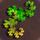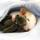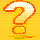General QuestionPrimes and Modular Arithmetic: Do any "complete" primes exist?

Asked by PhiNotPi (12677) March 2nd, 2012

A prime P > 2 is considered “complete” if for any whole number N < P, there is another whole number K (not necessarily < P) such that:
(K^2 + K)/2 mod P = N
Does any complete prime > 2 exist?

In the above equation, (K^2 + K)/2 is the formula for the Kth Triangular number.

For a more intuitive idea of what the whole idea of prime “completeness” is, imagine the following situation:

There is a prime number of people sitting in a circle, numbered 0 through P-1.
The first person in the circle receives \$1. The 1st person to his left also gets \$1. The 2nd person to that person’s left gets \$1, then the 3rd person to his left, and so on. If the prime is complete, then every single person will eventually end up with a lot of money. If it is not complete, then there will be a person who does not get any money.

The prime 3 is not complete, as you can see below in a list of who receives money:
person 0
person 1
person 0 (person 1+2=3 that has wrapped around the circle)
person 0 (person 3+3=6)
person 1 (person 6+4=10)
etc.
You can see that the last person, person 2, never gets any money.

Observing members: 0Composing members: 0((2*3)^2 + 2*3)/2 +2 = 23 = 7*3 + 2 = 2 mod 3.
In general, ((2*p)^2 + 2*p)/2 + n = 2*p^2 + p + n = n mod p.

I don’t think you asked the right question. Don’t you want to insure that (k^2 + k)/2 is in the orbit of the function given by f(0) = 1; f(n + 1) = f(n) + n – 1?

ratboy (15167)“Great Answer” (1) Flag as…No. There is no such p. Assume by contradiction there is a p satisfying this condition:

1) If k=l mod p then k^2+k=l^2+l mod p. Since p is odd, (k^2+k)/2=(l^2+l)/2 mod p.

2) If there is a k such that (k^2+k)/2=N mod p, you can assume 0<k<p because of (1).

3) Since for any 0<N<p there is a 0<k<p such that (k^2+k)/2=N mod p and we have exactly p-1 possibilities for N and p-1 possibilities for k, none of (k^2+k)/2 for 0<k<p can be divisible by p. However if we pick for k=p-1, the expression (k^2+k)/2 is divisible by p, which is a contradiction.

BonusQuestion (1483)“Great Answer” (1) Flag as…It appears that for any odd prime p, there are exactly (p+1)/2 different N’s, between 0 and p-1 that can be of the form (k^2+k)/2 mod p.

If that is something of any interest to you, I can post a proof so that you check my proof.

BonusQuestion (1483)“Great Answer” (1) Flag as…I am sure that @BonusQuestion has this fully worked out, but from an intuitive point of view, we know there are (p+1)/2 quadratic residues for prime p, so it seems reasonable that if we go from n^2 to (n^2+1)/2, we will again get (p+1)/2 residues.

LostInParadise (29287)“Great Answer” (1) Flag as…This is actually much easier to figure out than I first thought.
Suppose that (x^2 + x)/2 = n (mod p) has a solution for some x. Then x^2 + x =2n (mod p). If x=k is a solution, then another solution can be found by dividing x^2 + x – 2n by x – k. There will be exactly one n where x^2 + x -2n = (x – k)^2, namely k = -½ (mod p). There are thus (p – 1)/2 solutions that occur twice and one that occurs only once. giving (p+1)/2 solutions.

LostInParadise (29287)“Great Answer” (0) Flag as…@ratboy, in your equation, you say that
((2*3)^2 + 2*3)/2 +2 = 2 mod 3
Where did the +2 come from?

The equation need to be of the form
((K^2)+K)/2 = 2 mod 3

PhiNotPi (12677)“Great Answer” (0) Flag as…^^ Sleep typing—sorry.

ratboy (15167)“Great Answer” (0) Flag as…or